# Forth and back

Car goes from point A to point B at speed 78 km/h and back at 82 km/h. If went there and back at speed 81 km/h journey would take five minutes less. What is the distance between points A and B?

Result

x =  256.982 km

#### Solution:

x = 78a
x = 82b
2x = 81(a+b-5/60)

78a-x = 0
82b-x = 0
4860a+4860b-120x = 405

a = 369112 ≈ 3.294643
b = 351112 ≈ 3.133929
x = 1439156 ≈ 256.982143

Calculated by our linear equations calculator.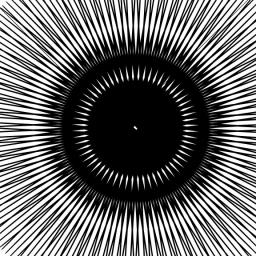Our examples were largely sent or created by pupils and students themselves. Therefore, we would be pleased if you could send us any errors you found, spelling mistakes, or rephasing the example. Thank you!

Leave us a comment of this math problem and its solution (i.e. if it is still somewhat unclear...):Be the first to comment!Tips to related online calculators
Looking for calculator of harmonic mean?
Looking for a statistical calculator?
Do you have a linear equation or system of equations and looking for its solution? Or do you have quadratic equation?
Do you want to convert velocity (speed) units?
Do you want to convert time units like minutes to seconds?

## Next similar math problems:

1. Two citiesThe car goes from city A to city B at an average speed of 70 km/h, back at an average speed of 50 km/h. If it goes to B and back at an average speed of 60 km/h, the whole ride would take 8 minutes less. What is the distance between cities A and B?
2. The truck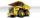The empty truck left the warehouse for goods at 10:00 and arrived at 11:30. At 2:00 pm, they loaded back on the same route. But it was a quarter slower than going to the warehouse. We know that on the way to the warehouse, the car was traveling at a speed
3. Two cyclists 2At the same time, two cyclists left the towns A and B at constant speeds. The first one going from town A to town B, and the second one from town B to town A. At one point of the trip they met. After they met, the first cyclist arrived at town B in 36min,
4. The touristThe tourist traveled 190km in 5 hours. Part of the journey passed at 5 km/h. The rest he went by bus at a speed of 60 km/h. How long has a bus gone?
5. Speed of car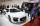The car went to a city that was 240 km away. If his speed increased by 8 km/h, it would reach the finish one hour earlier. Determine its original speed.
6. Car driver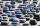The car driver is in town A and is scheduled to be in town B at a specified hour. If it travels at an average speed of 50km/h, it will arrive in city B 30 minutes later. But if he travels at an average speed of 70km/h, he would arrive half an hour earlier
7. Two portsFrom port A on the river, the steamer started at an average speed of 12 km/h towards port B. Two hours later, another steamer departed from A at an average speed of 20 km/h. Both ships arrived in B at the same time. What is the distance between ports A and
8. Two citiesThe distance between cities A and B is 132 km. At 9.00 am, the cyclist started the bike at an average speed of 24 km/h, and at 10.00 h started from the B cyclist at an average speed of 30 km/h. How long and far from A will they both meet?
9. The tourist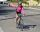The tourist came started from the hostel at an average speed of 5km/h. Half an hour later, the bicyclist started along the same route at a speed of 20km/h. How many minutes will a cyclist catch up and how many kilometers will he go?
10. Car and motorcyclistA car and a motorcyclist rode against each other from a distance of 190 km. The car drove 10km/h higher than the motorcyclist and started half an hour later. It met a motorcyclist in an hour and thirty minutes. Determine their speeds.
11. The escalator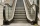I run up the escalator at a constant speed in the direction of the stairs and write down the number of steps A we climbed. Then we turn around and run it at the same constant speed in the opposite direction and write down the number of steps B that I climb
12. Bike rideMarek rode a bike ride. In an hour, John followed him on the same route by car, at an average speed of 72 km/h, and in 20 minutes he drove him. Will he determine the length of the way that Marek took before John caught up with him, and at what speed did Ma
13. Two trainsThrough the bridge, long l = 240m, the train passes through the constant speed at time t1 = 21s. A train running along the traffic lights at the edge of the bridge passes the same speed at t2 = 9s. a) What speed v did the train go? b) How long did it ta
14. Water current speed.Two cities along the river are 100 km apart. The powerboat downstream runs for 4 hours, upstream for 10 hours. Determines the river's current speed.
15. The train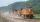The train passed the observer in the 20s and passed through a 1500m tunnel in 2 minutes. Calculate the speed (km/h) and train length.
16. Long bridge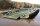Roman walked on the bridge. When he heard the whistle, he turned and saw running Kamil at the beginning of the bridge. If he went to him, they would meet in the middle of the bridge. Roman, however, rushed and so did not want to waste time returning 150m.Mr. Smith is on a road trip to a remote disc golf course 180km away. He travels part of the way at 100km/h along the highway, and part of the way at 50km/h along dirt roads. If it took 2 hours to get there, how many hours did he spend on dirt roads?